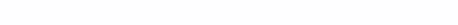# Deterministic Factor Analysis

Deterministic Factor Analysis is a method of researching the impact factors on effective indicator

It is assumed that the connection factors with effective indicator is functional in nature, which is expressed by a mathematical formula.

For example, the revenue of a logistics company can be represented as follows:it multiplicative model variant.

We want to track the change in revenue for the period: due to what the revenue in rubles changed in the reporting month in relation to the previous one. For this we will use integral way.

In the reporting month:
[B] revenue – 250 rubles,  price – 50 rubles / piece,  volume – 5 pcs;
in the previous month:
[A] revenue – 120 rubles,  price – 40 rubles / piece,  volume – 3 pcs.

This change can be represented by a rectangle:

0= 00,
1= (10) ∙ 0,
2= 0∙ (10),
3= (10) ∙ (10), 4= 0+ 1+ 2+ 3= 11

Change in revenue:
40 = 1+ 2+ 3
Change in revenue due to price:
1+ 3/ 2
Change in revenue due to volume:
2+ 3/ 2

Change in revenue: (250 – 120) = 130
Change in revenue due to price: (50 – 40) * 3 + [(50 – 40) * (5 – 3)]/ 2 = 30 + [10 * 2]/ 2 = 40
Change in revenue due to volume: 40 * (5 – 3) + [(50 – 40) * (5 – 3)]/ 2 = 80 + [10 * 2]/ 2 = 90
Let’s check: 130 = 40 + 90 (correct).

Revenue in the previous month was 120 rubles. A decrease in price by 10 rubles / piece increased revenue by 40 rubles, while an increase in volume by 2 pieces. increased revenue by 90 rubles. Revenue in the current month – 250 rubles.

For a two-dimensional case, you can use these formulas, but with an increase in the dimension of the model, it becomes problematic to choose formulas. We automate this process.

Let’s say that our model has become more complex now:In the reporting month:
[B] revenue – 250 rubles,  price – 5 rubles / kg,  volume – 5 pieces,  weight – 10 kg / piece;
in the previous month:
[A] revenue – 96 rubles,  price – 4 rubles / piece,  volume – 3 pieces  weight – 8 kg / piece.

Since the system has two states 0 and 1, then 4 must be split into 2 parts, where is the number of measurements.
So for 3 measurements you need 23 = 8 pieces.
Let’s make a table:

Where Δ = (1 − 0), Δ = (1 − 0),
1 – presence of Δ.
Delta (Δ) shows by what factor the change occurs.

The number of Δ in a row shows how many parts the figure must be divided into, for this it is necessary to display an additional column with the quantity of Δ (Δ).

The sums in the column , , ​​are the value of the influence of the factor.
As a result: A → 0+ + + = B

For our example:
Revenue in the previous month was 120 rubles. An increase in the price by 1 ruble / kg increased revenue by 36.3 rubles, while an increase in weight by 2 kg / piece. increased revenue by 36.3 rubles, also an increase in volume by 2 pcs. increased revenue by 81.3 rubles. Revenue in the current month – 250 rubles.

Now the code:

``````import numpy as np

from typing import *
from itertools import *

def factors_product(beg: List, end: List) -> List:

beg = np.array(beg)
end = np.array(end)
# (1) генерируем матрицу наличия Δ, (0, 0, 0) нам не нужна
sep = np.array(list(product([0, 1], repeat=len(beg))))[1:]
# (2) рассчитываем матрицу отклонений факторов + матрица начальных значений
delta = sep * (end-beg) + beg * (1-sep)
# (3) найдем Δ
k = sep.sum(1)
# (4) найдем произведение состояния факторов / Δ
m = (sep * (end-beg) + beg * (1-sep)).prod(1) / k
# (4) поместим m на соответствующие факторы и посчитаем суммы влияния
result = (sep.T * m).sum(1)
return result``````

Or return in one line:

``````def factors_product(beg: List, end: List) -> List:

beg = np.array(beg)
end = np.array(end)
sep = np.array(list(product([0, 1], repeat=len(beg))))[1:]

return (sep.T*((sep*(end-beg)+beg*(1-sep)).prod(1)/sep.sum(1))).sum(1)``````
``````# проверим нашу задачу
!pip install detfa

print(factors_product([4,3,8], [5,5,10]))
>> [36.33333333 81.33333333 36.33333333]``````